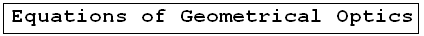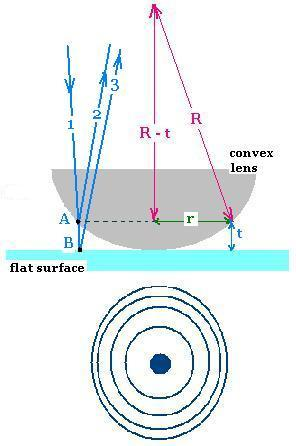Geometrical Optics: Geometrical Optics & Physics Optics. a Huygens Principle: Wavefronts. a Reflection: The first law of Geometrical Optics. a Refraction: The second law of Geometrical Optics: Snell's law. a Critical angle: Internal total reflection. a images formed by reflection Spherical concave mirrors. Mirror equation. a images formed by refraction Spherical convex mirrors .. a Fermat's principle: Fermat's principle for reflection and refraction.. a lenses: Thin lenses and Lens-makers' equation .. a prism: Prism, colors minimum deviation .. a Human eye: near point, far point, nearsightedness, farsightedness.. a Microscope: Microscope: simple, compound, and magnification.. a Telescope: Telescope: magnification.. a Resolution: optical microscope, electron microscope .. a dispersion: dispersion, more about the refractive index, and colors of a prism .. a parallel sheet: deviation by a parallel sheet .. a thin films : thin films and Newton's rings.. a Newton's rings: thin films and Newton's rings.. a Descartes rainbow: Primary and secondary rainbows .. a Some applications More fun with Optics .. a Optics calculator: Optics calculator: all the related calculations .. a home The fundamental, and just this .. a scientificSentence a __________________ a

Geometrical Optics: Applications: Newton's rings
Optics -     Geometrical Optics..

### 1. Newton's ringsThe device to prodice Newton's rings contains A flat surface, a convex lens (spherical surface) with an air wedge formed between them. The lens is illuminated with normally incident monochromatic light (ray 1). The circular fringes viewed are concentric circles around the point of contact with the flat surface.

R is the radius of curvature of the lens, T is the thickness of the lens where we choose r.

Pythagorean theorem gives:
r2 + (R - t)2 = R2

Therefore
r2 + t2 - 2 R t = 0

Because it is small, we neglect the term t2, hence:
r2 = 2 R t , or
t = r2/2R

t = r2/2R    (1)

At the point A, th reflected ray does not undergo a phase change, but at the point B the reflected ray 2 undergoes a π phase change.

Therefore
Δφ = φ3 - φ2 = (φB + φ(t)) - φA

The path difference between reflected rays is δ = 2t
φ(t) = 2 π δ/λ = 4 π t/λ

Hence:
Δφ = (π + (4πt/λ)) - o =
π(1 + (4t/λ))

1.The bright fringes corresponds the constructive interferences and a phase difference of 2 m π. That is:
2 m π = π(1 + (4t/λ))

Hence:
2 m = (1 + (4t/λ)), or:
t = (2 m - 1)λ/4

t = (2 m - 1)λ/4    (2)

2. The dark fringes corresponds the desstructive interferences and a phase difference of (2 m + 1) π. That is:
(2 m + 1) π = π(1 + (4t/λ))

Hence:
2 m = 4t/λ), or:
t = mλ/2

From equation (1), the radius r of the m-th ring corresponds to the thickness of the air wedge t :

r = (2 R t )1/2

The bright m-th ring corresponds to
r = (2 R t )1/2 = [R (m - 1/2)λ]1/2    (3)

The dark m-th ring corresponds to
r = (2 R t )1/2 = (R mλ)1/2    (4)

The bright m-th ring corresponds to:
r = [R (m - 1/2)λ]1/2
The dark m-th ring corresponds to:
r = (R mλ)1/2

Remarks:

1. The index of refraction of the lens has no importante.
2. The index of refraction of air is n = 1 that gives:
λ (in air) = λ(in vacuum) = λ

### 2. Example

If the incident light has a lambda; = 500 nm, and R = 2.00 m, what is the radius of the 4th bright ring ?

r = [R (m - 1/2)λ]1/2 =
[2.00 (4 - 1/2)5 x 10 -7>]1/2 =
18.71 x 10 -4> m = 1.9 mm.

 @import url(http://www.google.com/cse/api/branding.css);Custom Search © 2009. The scientificsentence . All rights reserved.